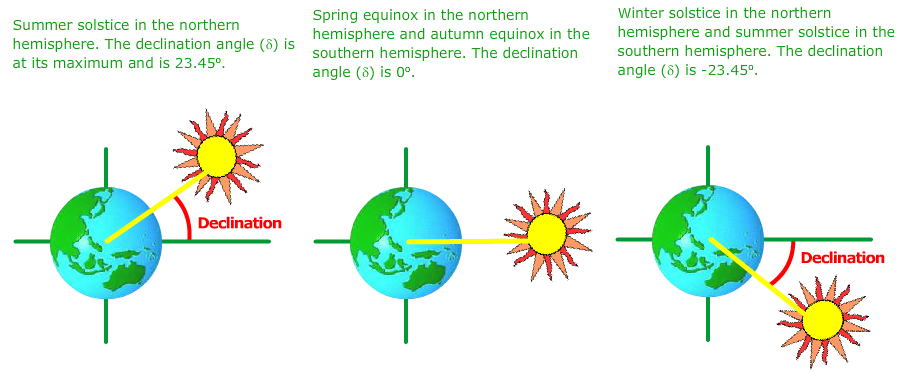# Declination Angle

The declination angle, denoted by δ, varies seasonally due to the tilt of the Earth on its axis of rotation and the rotation of the Earth around the sun. If the Earth were not tilted on its axis of rotation, the declination would always be 0°. However, the Earth is tilted by 23.45° and the declination angle varies plus or minus this amount. Only at the spring and fall equinoxes is the declination angle equal to 0°. The rotation of the Earth around the sun and the change in the declination angle is shown in the animation below.Animation showing how the tilt angle changes from the summer solstice in the northern hemisphere (or winter in the southern hemisphere) to the northern hemisphere winter solstice (summer in the south).

The declination of the sun is the angle between the equator and a line drawn from the centre of the Earth to the centre of the sun. The seasonal variation of the declination angle is shown in the animation below.Despite the fact that the Earth revolves around the sun, it is simpler to think of the sun revolving around a stationary Earth. This requires a coordinate transformation. Under this alternative coordinate system, the sun moves around the Earth.The declination angle can be calculated by the equation 1:

## Declination Angle

where d is the day of the year with Jan 1 as d = 1

The declination is zero at the equinoxes (March 22 and September 22), positive during the northern hemisphere summer and negative during the northern hemisphere winter. The declination reaches a maximum of 23.45° on June 22 (summer solstice in the northern hemisphere) and a minimum of -23.45° on December 21-22 (winter solstice in the northern hemisphere). In the equation above, the +10 comes from the fact that the winter solstice occurs before the start of the year. The equation also assumes that the suns orbit is a perfect circle and the factor of 360/365 converts the day number to a position in the orbit.

### Alternate Formulations

The declination angle can also be defined in other ways. The derivation of the declination angle assumes a circular orbit and gives the following result:

$$sin\ \delta=\sin{\left({-23.45}^0\right)}\cos{\left[\frac{360}{365}\left(d+10\right)\right]}$$

In radians, δ ≈ sin δ, for small values of δ so the derivation reduces to the equation given in the previous section:

## Declination Angle

The difference between the two equations is very small (< 0.3°), whereas the error to the true declination (e.g. the PSA algorithm below) is up to 1°. Thus the simpler equation is preferred since it is equally accurate.

The equations:

## Declination Angle (alternate)

are also often used in the literature. They are referred to the equinoxes instead so use sin instead of cos.

Finally, there are many algorithms for more accurate determinations of declination angle to account for the elliptic and yearly movement of the earth's orbit. These are only needed for concentrators that require more accurate tracking of the sun.

For instance the SPA algorithm 2(http://www.psa.es/sdg/sunpos.htm) uses:

dOmega=2.1429-0.0010394594*dElapsedJulianDays;

dMeanLongitude = 4.8950630+ 0.017202791698*dElapsedJulianDays;

// Radians dMeanAnomaly = 6.2400600+ 0.0172019699*dElapsedJulianDays;

dEclipticLongitude = dMeanLongitude + 0.03341607*sin( dMeanAnomaly ) + 0.00034894*sin( 2*dMeanAnomaly )-0.0001134 -0.0000203*sin(dOmega);

dEclipticObliquity = 0.4090928 - 6.2140e-9*dElapsedJulianDays +0.0000396*cos(dOmega);

dSin_EclipticLongitude= sin( dEclipticLongitude );

dY = cos( dEclipticObliquity ) * dSin_EclipticLongitude;

dX = cos( dEclipticLongitude );

dRightAscension = atan2( dY,dX ); if( dRightAscension < 0.0 ) dRightAscension = dRightAscension + twopi;

dDeclination = asin( sin( dEclipticObliquity )*dSin_EclipticLongitude );

dElapsedJulian days is the number of days since January 1, 2000 and dDeclination is the resulting declination.

The various ways of calculating the declination angle are graphed below using declination.py python code.<